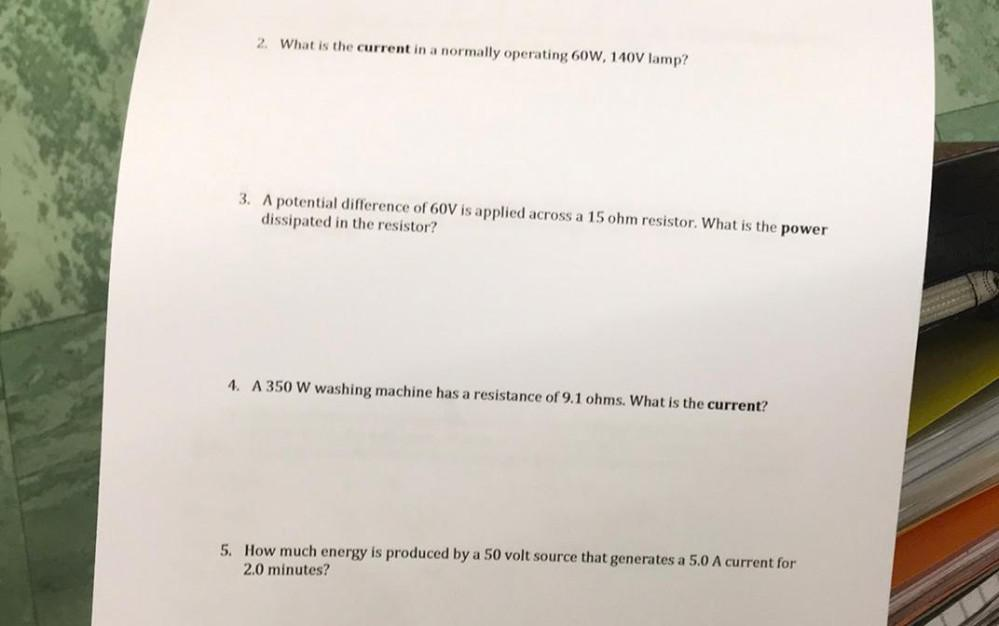Question:

# (2) What is the current in a normally operating 60W, 140V lamp? (3) A potential difference of 60V is applied across a 15 ohm resistor. What is the power dissipated in the resistor? (4) A 350 W washin(2) What is the current in a normally operating 60W, 140V lamp? (3) A potential difference of 60V is applied across a 15 ohm resistor. What is the power dissipated in the resistor? (4) A 350 W washing machine has a resistance of 9.1 ohms. What is the current? (5) How much energy is produced by a 50 volt source that generates a 5.0 A current for 2.0 minutes?